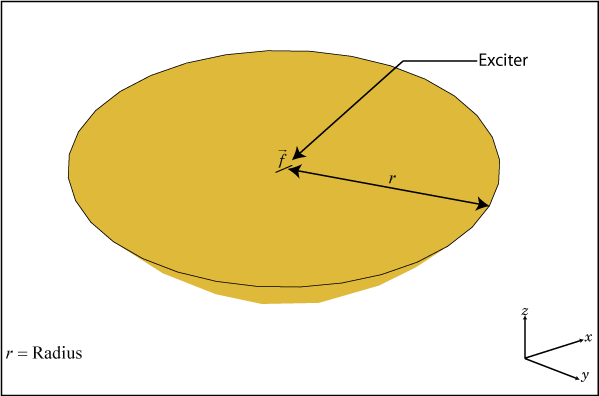Main Content

# reflectorParabolic

Create parabolic reflector antenna

## Description

The `reflectorParabolic` object creates a parabolic reflector antenna. Parabolic reflector antennas are electrically large structures and are at least 10 wavelengths in diameter. These reflectors are used in TV antennas and satellite communications, for example.## Creation

### Syntax

``ant = reflectorParabolic``
``ant = reflectorParabolic(Name,Value)``

### Description

example

````ant = reflectorParabolic` creates a dipole-fed parabolic reflector antenna. The default antenna exciter operates at 10 GHz. The reflector is 10λ in diameter, where λ corresponds to the value of wavelength.```
````ant = reflectorParabolic(Name,Value)` sets properties using one or more name-value pairs. For example, `ant = reflectorParabolic('FocalLength',0.5)` creates a parabolic reflector antenna of focal length 0.5 meters.```

## Properties

expand all

Antenna type used as an exciter, specified as any single-element antenna object. Except reflector and cavity antenna elements, you can use any of the antenna elements or array elements in the Antenna Toolbox™ as an exciter.

Example: `'Exciter',horn`

Example: `ant.Exciter = horn`

Example: ```ant.Exciter = linearArray('patchMicrostrip')```

Radius of the parabolic reflector, specified as a positive scalar integer in meters.

Example: `'Radius',0.22`

Example: `ant.Radius = 0.22`

Data Types: `double`

Focal length of the parabolic dish, specified as a positive scalar integer in meters.

Example: `'FocalLength',0.0850`

Example: `ant.FocalLength = 0.0850`

Data Types: `double`

Signed distance from the focus of the parabolic dish, specified as a three-element vector in meters. By default, the antenna exciter is at the focus of the parabola. Using the `FeedOffset` property, you can place the exciter anywhere on the parabola.

Example: `'FeedOffset',[0.0850 0 0]`

Example: `ant.FeedOffset = [0.0850 0 0]`

Data Types: `double`

Type of the metal used as a conductor, specified as a metal material object. You can choose any metal from the `MetalCatalog` or specify a metal of your choice. For more information, see `metal`. For more information on metal conductor meshing, see Meshing.

Example: ```m = metal('Copper'); 'Conductor',m```

Example: ```m = metal('Copper'); ant.Conductor = m```

Lumped elements added to the antenna feed, specified as a lumped element object handle. For more information, see `lumpedElement`.

Example: `'Load',lumpedelement`. `lumpedelement` is the object handle for the load created using `lumpedElement`.

Example: ```ant.Load = lumpedElement('Impedance',75)```

Tilt angle of the antenna, specified as a scalar or vector with each element unit in degrees. For more information, see Rotate Antennas and Arrays.

Example: `'Tilt',90`

Example: `ant.Tilt = 90`

Example: `'Tilt',[90 90]`,`'TiltAxis',[0 1 0;0 1 1]` tilts the antenna at 90 degrees about the two axes defined by the vectors.

Note

The `wireStack` antenna object only accepts the dot method to change its properties.

Data Types: `double`

Tilt axis of the antenna, specified as:

• Three-element vector of Cartesian coordinates in meters. In this case, each coordinate in the vector starts at the origin and lies along the specified points on the X-, Y-, and Z-axes.

• Two points in space, each specified as three-element vectors of Cartesian coordinates. In this case, the antenna rotates around the line joining the two points in space.

• A string input describing simple rotations around one of the principal axes, 'X', 'Y', or 'Z'.

For more information, see Rotate Antennas and Arrays.

Example: `'TiltAxis',[0 1 0]`

Example: `'TiltAxis',[0 0 0;0 1 0]`

Example: `ant.TiltAxis = 'Z'`

Note

The `wireStack` antenna object only accepts the dot method to change its properties.

Data Types: `double`

## Object Functions

 `show` Display antenna or array structure; display shape as filled patch `axialRatio` Axial ratio of antenna `beamwidth` Beamwidth of antenna `charge` Charge distribution on metal or dielectric antenna or array surface `current` Current distribution on metal or dielectric antenna or array surface `design` Design prototype antenna or arrays for resonance at specified frequency `efficiency` Radiation efficiency of antenna `EHfields` Electric and magnetic fields of antennas; Embedded electric and magnetic fields of antenna element in arrays `impedance` Input impedance of antenna; scan impedance of array `mesh` Mesh properties of metal or dielectric antenna or array structure `meshconfig` Change mesh mode of antenna structure `optimize` Optimize antenna or array using SADEA optimizer `pattern` Radiation pattern and phase of antenna or array; Embedded pattern of antenna element in array `patternAzimuth` Azimuth pattern of antenna or array `patternElevation` Elevation pattern of antenna or array `returnLoss` Return loss of antenna; scan return loss of array `sparameters` S-parameter object `vswr` Voltage standing wave ratio of antenna

## Examples

collapse all

Create and view a default parabolic reflector antenna.

`ant = reflectorParabolic`
```ant = reflectorParabolic with properties: Exciter: [1x1 dipole] Radius: 0.1500 FocalLength: 0.0750 FeedOffset: [0 0 0] Tilt: 0 TiltAxis: [1 0 0] Load: [1x1 lumpedElement] ```
`show(ant)`Plot the radiation pattern of the parabolic reflector at 10 GHz.

`pattern(ant,10e9)`Create a circular array of equiangular spiral antennas.

`circA = circularArray('Element',spiralEquiangular,'Radius',0.1);`

Create a parabolic reflector-backed antenna.

`ant = reflectorParabolic('Exciter',circA)`
```ant = reflectorParabolic with properties: Exciter: [1x1 circularArray] Radius: 0.1500 FocalLength: 0.0750 FeedOffset: [0 0 0] Tilt: 0 TiltAxis: [1 0 0] Load: [1x1 lumpedElement] ```
`show(ant)`## See Also

### Topics

Introduced in R2018b

## Support

#### Hybrid Beamforming for Massive MIMO Phased Array Systems

Download the white paper